《深度学习入门：基于Python的理论与实现》第三章阅读笔记

3 神经网络

3.1 从感知机到神经网络

3.1.1 神经网络的例子

3.1.2 复习感知机

3.1.3 激活函数登场

3.2 激活函数

3.2.1 sigmoid函数

3.2.2 阶跃函数的实现

3.2.3 阶跃函数的图像

3.2.4 sigmoid函数的实现

3.2.5 sigmoid函数和阶跃函数的比较

3.2.6 非线性函数

3.2.7 ReLU函数

3.3 多维数组的运算

3.3.1 多维数组

3.3.2 矩阵乘法

3.3.3 神经网络的内积

3.4 3层神经网络的实现

3.4.1 符号确认

3.4.2 各层间信号传递的实现

3.4.3 代码实现小结

3.5 输出层的设计

3.5.1 恒等函数和softmax函数

3.5.2 实现softmax函数时的注意事项

3.5.3 softmax函数的特征

3.5.4  输出层的神经元数量

3.6 手写数字识别

3.6.1 MNIST数据集

3.6.2 神经网络的推理处理

3.6.3 批处理

3 神经网络

3.1 从感知机到神经网络

3.1.1 神经网络的例子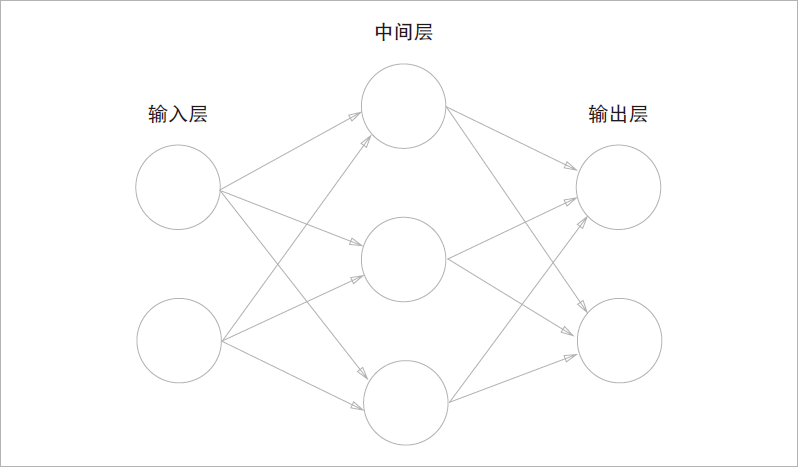3.1.2 复习感知机（注意上图没有画出偏置项）

3.2 激活函数

3.2.2 阶跃函数的实现

def step_function(x):
y = x > 0
return y.astype(np.int)

3.2.3 阶跃函数的图像

import numpy as np
import matplotlib.pyplot as plt

def set_function(x):
return np.array(x > 0, dtype=np.int)

x = np.arange(-5.0, 5.0, 0.1)
y = set_function(x)
plt.plot(x, y)
plt.ylim(-0.1, 1.1) # 指定y轴范围
plt.show()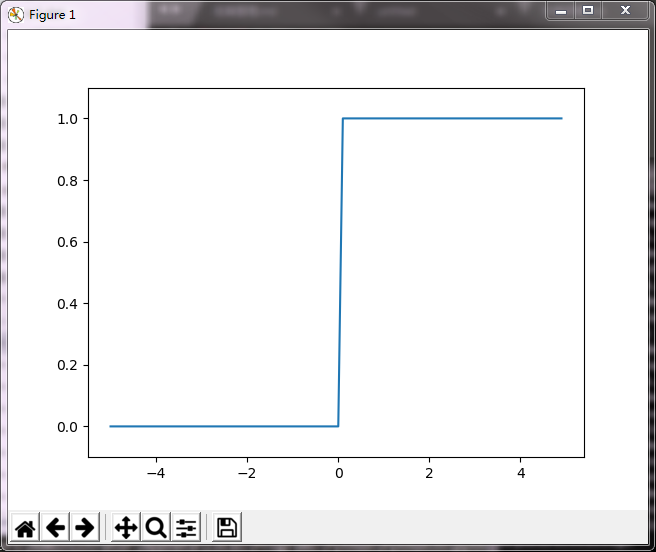3.2.4 sigmoid函数的实现

def sigmoid(x):
return 1 / (1 + np.exp(-x))

import numpy as np
import matplotlib.pyplot as plt

def sigmoid(x):
return 1 / (1 + np.exp(-x))

x = np.arange(-5.0, 5.0, 0.1)
y = sigmoid(x)
plt.plot(x, y)
plt.ylim(-0.1, 1.1) # 指定y轴范围
plt.show()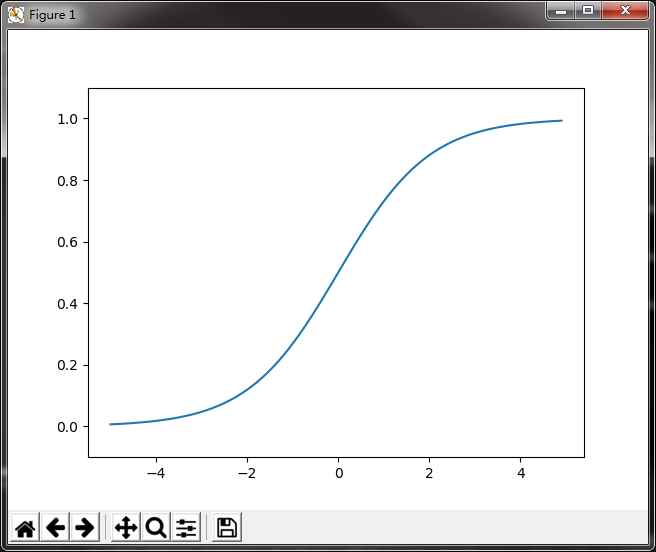3.2.5 sigmoid函数和阶跃函数的比较

sigmoid较阶跃函数有更平滑的特性，对神经网络的学习有重要意义（梯度下降）。

3.2.7 ReLU函数

import numpy as np
import matplotlib.pyplot as plt

def relu(x):
return np.maximum(0, x)

x = np.arange(-5.0, 5.0, 0.1)
y = relu(x)
plt.plot(x, y)
plt.ylim(-0.1, 5.1) # 指定y轴范围
plt.show()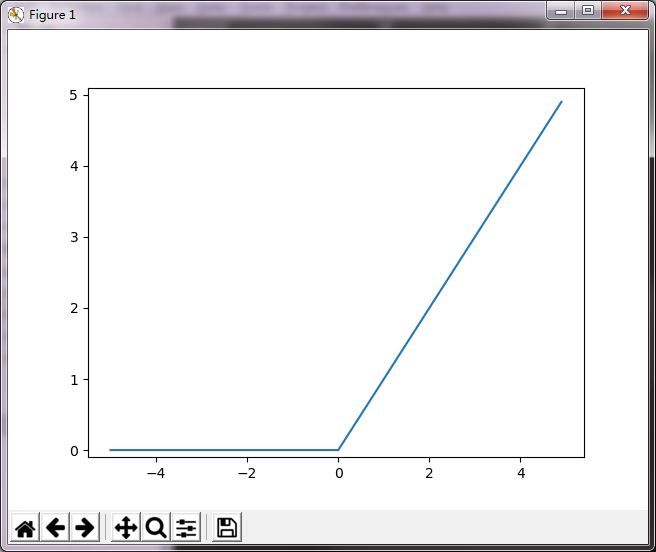3.3 多维数组的运算

3.3.1 多维数组

a = np.array([[1.1, 1.2, 1.3], [2.1, 2.2, 2.3], [3.1, 3.2, 3.3]])
print(a)
np.ndim(a) # 返回a的纬度
a.shape # tuple类型，返回a的形状

3.3.2 矩阵乘法

np.dot(A, B)

3.3.3 神经网络的内积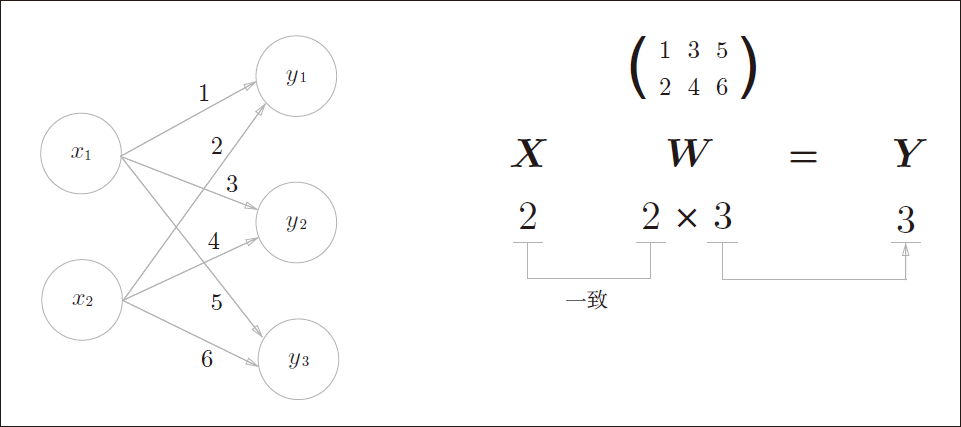X = np.array([1, 2])
W = np.array([[1, 3, 5], [2, 4, 6]])
Y = np.dot(X, W)

3.4 3层神经网络的实现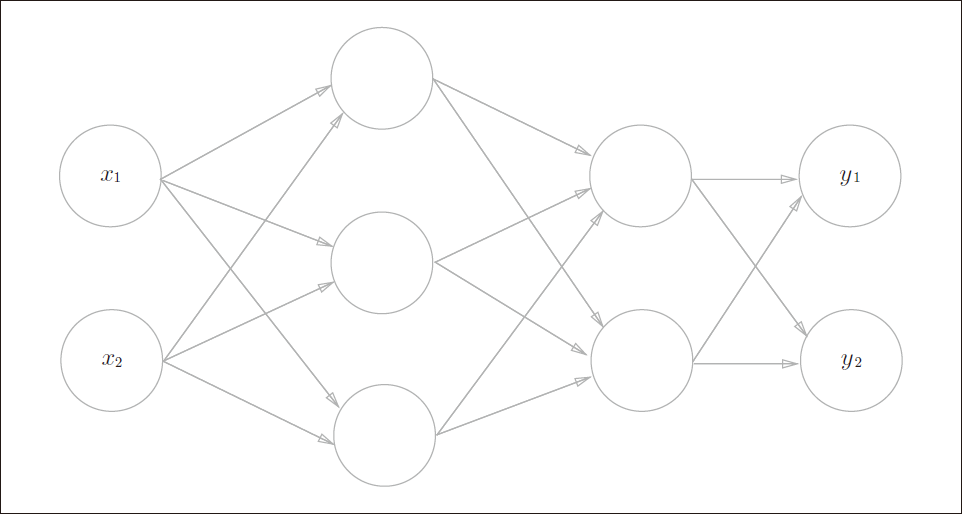3层神经网络：输入层（第0层）有两个神经元，第一层隐藏层（第1层）有三个神经元，第二层隐藏层（第2层）有两个神经元，输出层（第3层）有两个神经元。

3.4.1 符号确认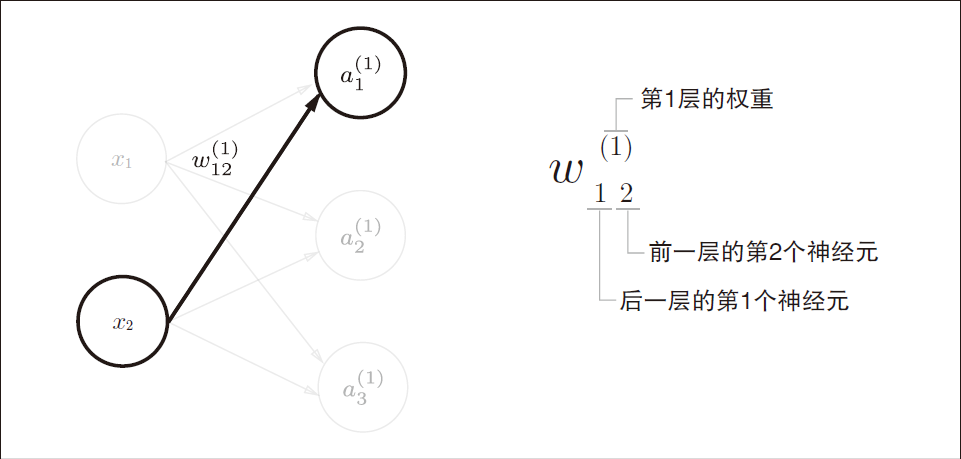3.4.2 各层间信号传递的实现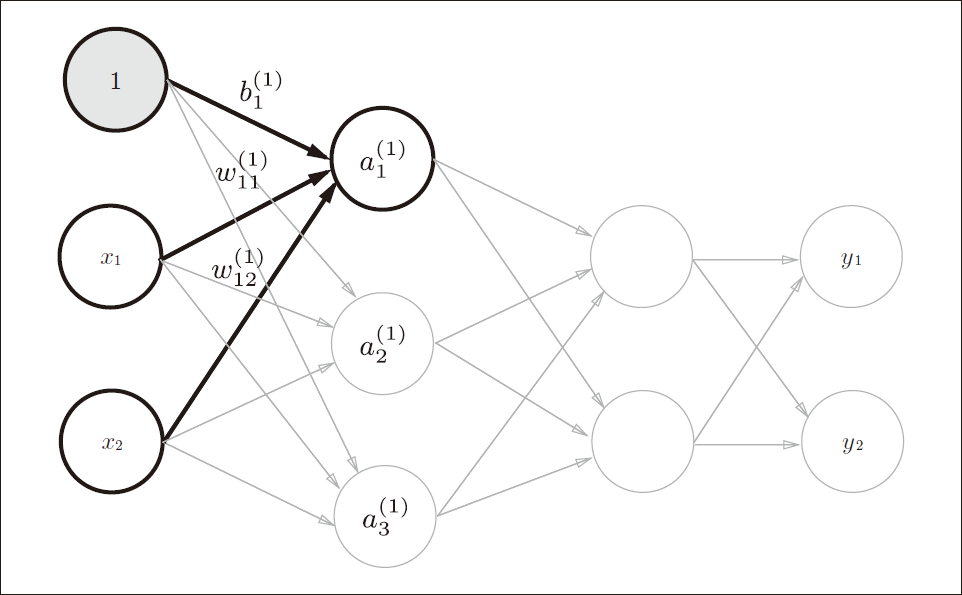,

,

X = np.array([1.0, 0.5])
W1 = np.array([[0.1, 0.3, 0.5], [0.2, 0.4, 0.6]])
B1 = np.array([0.1, 0.2, 0.3])

A1 = np.dot(X, W1) + B1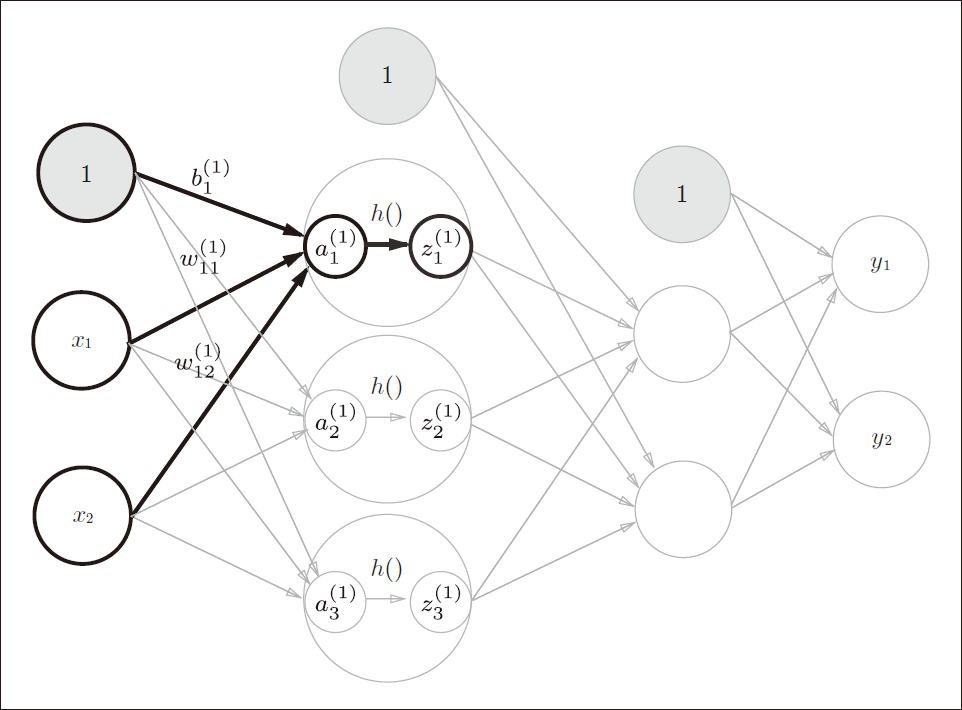Z1 = sigmoid(A1)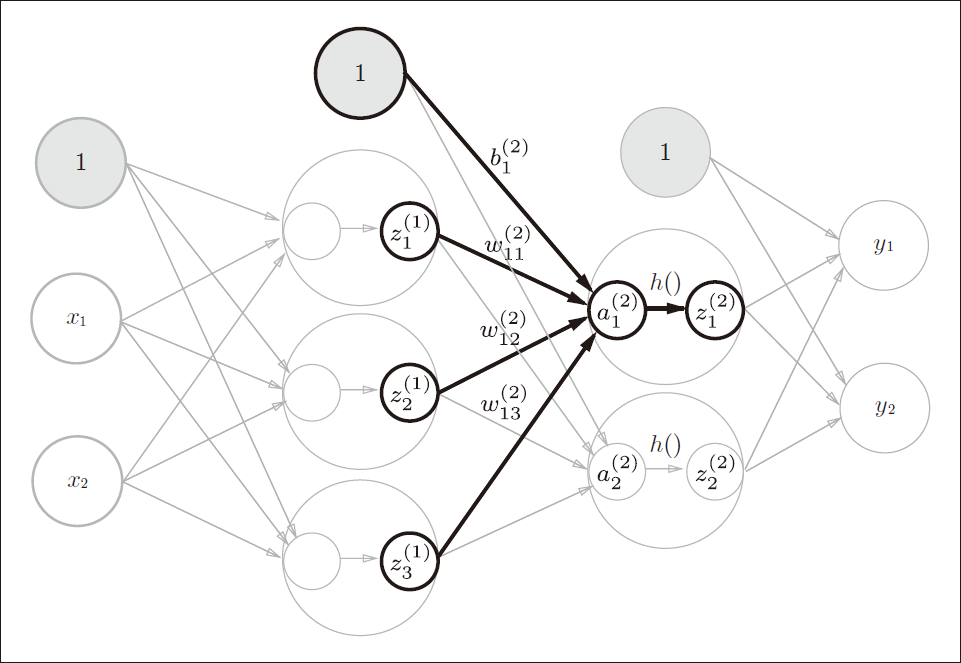W2 = np.array([[0.1, 0.4], [0.2, 0.5], [0.3, 0.6]])
B2 = np.array([0.1, 0.2])

A2 = np.dot(Z1, W2) + B2
Z2 = sigmoid(A2)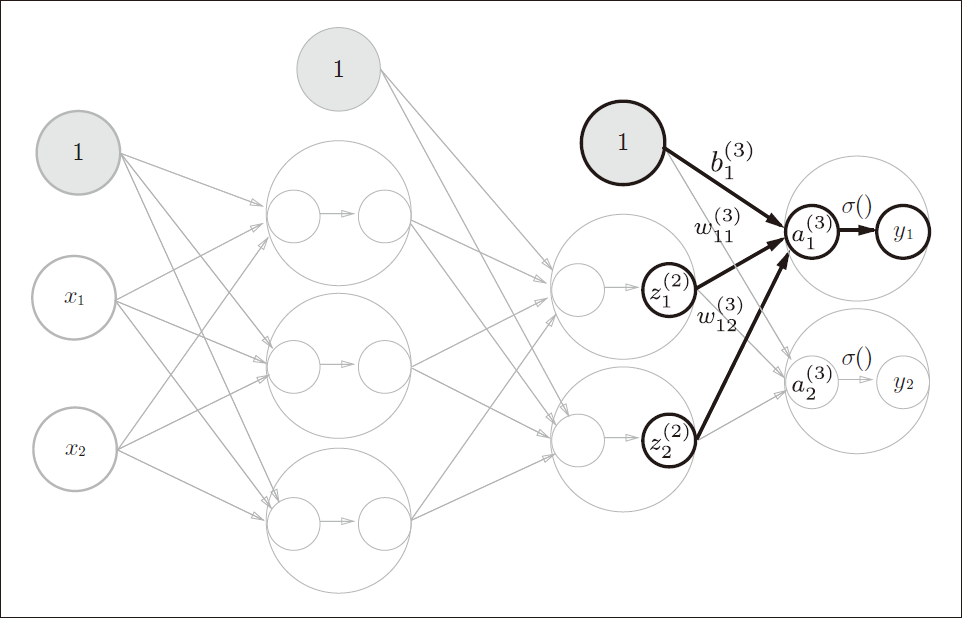def identity_function(x):
return x

W3 = np.array([[0.1, 0.3], [0.2, 0.4]])
B3 = np.array([0.1, 0.2])

A3 = np.dot(Z2, W3) + B3
Y  = identity_function(A3) # 或 Y = A3

3.4.3 代码实现小结

import numpy as np

def identity_function(x):
return x

def sigmoid(x):
return 1 / (1 + np.exp(-x))

def init_network():
network = {}
network['W1'] = np.array([[0.1, 0.3, 0.5], [0.2, 0.4, 0.6]])
network['b1'] = np.array([0.1, 0.2, 0.3])
network['W2'] = np.array([[0.1, 0.4], [0.2, 0.5], [0.3, 0.6]])
network['b2'] = np.array([0.1, 0.2])
network['W3'] = np.array([[0.1, 0.3], [0.2, 0.4]])
network['b3'] = np.array([0.1, 0.2])

return network

def forward(network, x):
W1, W2, W3 = network['W1'], network['W2'], network['W3']
b1, b2, b3 = network['b1'], network['b2'], network['b3']

a1 = np.dot(x , W1) + b1
z1 = sigmoid(a1)
a2 = np.dot(z1, W2) + b2
z2 = sigmoid(a2)
a3 = np.dot(z2, W3) + b3
y  = identity_function(a3)

return y

network = init_network()
x = np.array([1.0, 0.5])
y = forward(network, x)
print(y) # [0.31682708 0.69627909]


3.5 输出层的设计

3.5.1 恒等函数和softmax函数

softmax的分子是输入信号的指数函数，分母是所有输入信号的指数的和。

def softmax(a):
exp_a = np.exp(a)
sum_exp_a = np.sum(exp_a)
y = exp_a / sum_exp_a

return y

3.5.2 实现softmax函数时的注意事项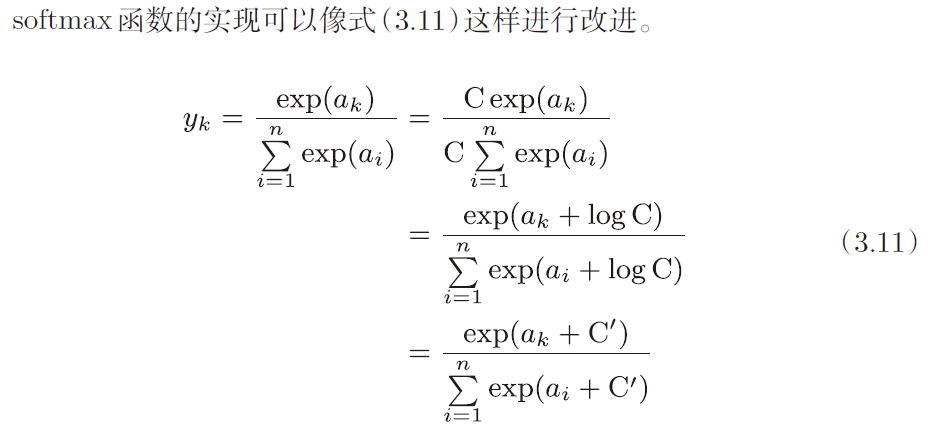def softmax(a):
c = np.max(a)
exp_a = np.exp(a - c) # 溢出对策
sum_exp_a = np.sum(exp_a)
y = exp_a / sum_exp_a

return y

3.5.3 softmax函数的特征

a = np.array([0.3, 2.9, 4.0])
y = softmax(a)
print(y) # [0.01821127 0.24519181 0.73659691]

3.6 手写数字识别

3.6.1 MNIST数据集

MNIST数据集是右0到9的数字图像构成的。训练图像有6万张，测试图像有1万张，这些图像可用于学习和推理。

MNIST的图像数据是28px * 28px的灰度图像（1通道），各个像素的取值在0到255之间。每个图像数据都相应地标有该图实际对应的数字标签。

from dataset.mnist import load_mnist

(x_train, t_train), (x_test, t_test) = load_mnist(flatten=True, normalize=False)

print(x_train.shape) # (60000, 784)
print(t_train.shape) # (60000,)
print(x_test.shape)	 # (10000, 784)
print(t_test.shape)  # (10000,)

# pip install Pillow

import numpy as np
from PIL import Image

def img_show(img):
pil_img = Image.fromarray(np.uint8(img))
pil_img.show()

(x_train, t_train), (x_test, t_test) = load_mnist(flatten=True, normalize=False)
img = x_train
label = t_train
print(label)				# 5

print(img.shape)			# (784,)
img = img.reshape(28, 28)
print(img.shape)			# (28, 28)

img_show(img)



3.6.2 神经网络的推理处理

2个隐藏层，第一个隐藏层有50个神经元，第二个隐藏层有100个神经元。（这里50和100可以换成其它值，需要在数组维度上保持一致即可）

def get_data():
(x_train, t_train), (x_test, t_test) = load_mnist(flatten=True, normalize=True, one_hot_label=False)
return x_test, t_test

# init_network()会读入保存在pickle文件sample_weight.pkl中学习到的权重参数
# 这个文件中以字典变量的形式保存了权重和偏置参数
def init_network():
with open("sample_weight.pkl", "rb") as f:

return network

def predict(network, x):
W1, W2, W3 = network['W1'], network['W2'], network['W3']
b1, b2, b3 = network['b1'], network['b2'], network['b3']

a1 = np.dot(x,  W1) + b1
z1 = sigmoid(a1)
a2 = np.dot(z1, W2) + b2
z2 = sigmoid(a2)
a3 = np.dot(z2, W3) + b3
y  = softmax(a3)

return y

x, t = get_data()
network = init_network()

accuracy_cnt = 0
for i in range(len(x)):
y = predict(network, x[i])
p = np.argmax(y)
if p == t[i]:
accuracy_cnt += 1

print("accuracy: " + str(float(accuracy_cnt) / len(x)))

3.6.3 批处理

x, t = get_data()
network = init_network()

batch_size = 100 # 批数量
accuracy_cnt = 0

for i in range(0, len(x), batch_size):
x_batch = x[i:i+batch_size]
y_batch = predict(network, x_batch)
p = np.argmax(y_batch, axis=1)
accuracy_cnt += np.sum(p == t[i:i+batch_size])

print("accuracy: " + str(float(accuracy_cnt) / len(x)))

本章所学内容

• 神经网络中的激活函数使用平滑变化的sigmoid函数或ReLU函数
• 通过巧妙地使用NumPy多维数组，可以高效地实现神经网络
• 机器学习的问题大体上可以分为回归问题和分类问题
• 关于输出层的激活函数，回归问题中一般用恒等函数，分类问题中一般用softmax函数
• 分类问题中，输出层的神经元的数量设置为要分类的类别数
• 输入数据的集合称为批。通过以批为单位进行推理处理，能够实现高速的运算。